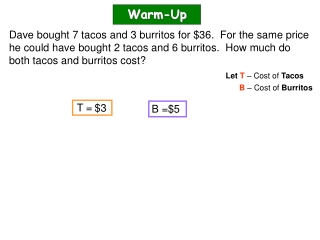DownloadDownload PresentationWarm-Up

# Warm-Up

Download Presentation## Warm-Up

- - - - - - - - - - - - - - - - - - - - - - - - - - - E N D - - - - - - - - - - - - - - - - - - - - - - - - - - -
##### Presentation Transcript

1. Warm-Up Dave bought 7 tacos and 3 burritos for \$36. For the same price he could have bought 2 tacos and 6 burritos. How much do both tacos and burritos cost? LetT – Cost of Tacos B – Cost of Burritos T = \$3 B =\$5

2. CA Standards 9.0: Students solve a system of two linear equations in two variables algebraically and are able to interpret the answer graphically. Students are able to solve a system of two linear inequalities in two variables and to sketch the solution sets. Objective: (1) Students will solve word problems using systems of equations. Agenda: 12/06/2011 1.) Warm-up 2.) Lesson: Word Problems – Cost Quantity (ppt.) 3.) Class/Home Work One (1) Assignment 4.) STAY ON TASK!!!!

3. 8.4 Word Problems – Cost/Quantity Notes: Word Problems Type: Cost Quantity Ex 1) A local record company sells CDs for \$15 and DVDs for \$20. If they made \$170 while selling 10 items, how many of each did they sell? LetC – # of CDs 15C + 20D = 170 15C + 20D = 170 D – # of DVDs –15D = -150 -15 -15C = 10 C + D 5D + 0 =20 5 5 4 D = C + D = 10 C + (4) = 10 -4 -4 C = 6

4. 8.4 Word Problems – Cost/Quantity Try this one: Ex 2) A local record company sells CDs for \$10 and DVDs for \$18. If they made \$188 while selling 14 items, how many of each did they sell? LetC – # of CDs 10C + 18D = 188 10C + 18D = 188 D – # of DVDs –10D = -140 -10 -10C = 14 C + D 8D + 0 =48 8 8 6 D = C + D = 14 C + (6) = 14 -6 -6 C = 8

5. 8.4 Word Problems – Cost/Quantity Notes: Word Problems Type: Cost Quantity Ex 3) Burritos cost \$5 and tacos cost \$3. How many of each did I buy if I got 8 items for \$36? LetB – # of Burritos 5B + 3T = 36 5B + 3T = 36 T – # of Tacos – 5T = -40 -5B = 8 B + T -5 – 2T 0 =-4 -2 -2 2 T = B + T = 8 B + (2) = 8 -2 -2 B = 6

6. 8.4 Word Problems – Cost/Quantity Try this one !!!!! Ex 4) Burritos cost \$3 and tacos cost \$1. How many of each did I buy if I got 21 items for \$53? LetB – # of Burritos 3B + 1T = 53 3B + 1T = 53 T – # of Tacos – 3T = -63 -3B = 21 B + T -3 – 2T 0 =-10 -2 -2 5 T = B + T = 21 B + (5) = 21 -5 -5 B = 16

7. 8.4 Word Problems – Cost/Quantity Notes: Word Problems Type: Cost Quantity Ex 5) A child’s ticket costs \$8 for admission into the movie theater. Adult tickets cost \$12. How many of each did a family get if they spent \$48 for 5 tickets? LetC – # of Child tickets 8C + 12A = 48 8C + 12A = 48 A – # of Adult tickets – 8A = -40 -8C = 5 -8 C + A 4A 0 + =8 4 4 2 A = C + A = 5 C + (2) = 5 -2 -2 C = 3